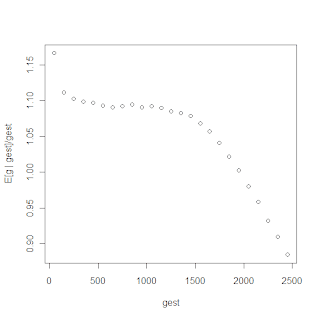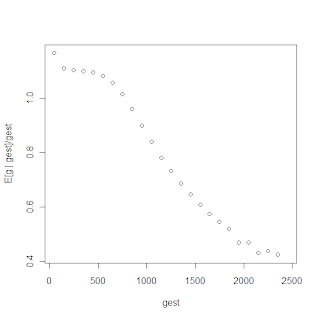## October 13, 2008

### Estimating TMRCA for a pair of Y-STR haplotypes using Average Squared Distance (ASD) cont'd

This is a continuation of On the use of average squared distance (ASD) to estimate the time to most recent common ancestor (TMRCA) of a pair of Y-STR haplotypes inspired by James Heald's interesting comments. I suggest that you read that post first if you want to make sense of this one.

Summary of first post

In the first post I studied via simulation, the distribution P(gest | g) where g is the real TMRCA, and gest = ASD(hta, htb)/2μest is its estimate via average squared distance of two haplotypes hta and htb.

It was shown that the expected value E[gest | g] = g, and also that gest varies by quite a lot around g.

E[g | gest]

What can we say about E(g | gest), i.e. the expected value of the TMRCA if we have an estimate of its age? In other words, the expected real age, given its apparent age.

To study this, I sample g from a prior distribution P(g) and determine for each sample (via simulation) the corresponding gest. Thus, for each gest I have a set {g1, g2, ..., gn} (real ages), whose apparent age is gest.

Then:

E[g | gest] = mean{g1, g2, ..., gn}

Figuring out the prior distribution P(g) is of course the real trick. Bruce Walsh suggests (Genetics 158: 897–912) using p(t) = λexp(-λt) with λ=1/Ne, where t is the TMRCA and Νe is the effective population size. He cites an estimate for this Ne=5000 for humans, and discovers that the posterior distribution of t is not very sensitive to the prior.

Various kinds of belief or evidence can be incorporated in the prior. For example, we can set P(g)=0 if g>2,500 since two Y-chromosomes cannot have an MRCA older than "Y-chromosome Adam" -- if we are convinced that 2,500 generations is an upper limit on the age of Y-chromosome Adam.

But, if we are comparing Y-chromosomes of patrilineal descendants of a known founder (e.g., Genghis Khan), then we can set P(g) = 0 if g>40. Conversely, if we are comparing an R1a and R1b haplotype then we can set P(g) = 0 for g less than e.g., 160 generations, since the MRCA of R1a and R1b must be older than the time in which R1a1 has been detected in ancient DNA.

The point is that different real ages can lead to the same observed apparent age. To go from the apparent age to the real one, we can use prior information about it, i.e., the P(g) distribution.

It is important to note that P(g) can be seen as our prior belief about the distribution of g, that is: given two Y-chromosomes, what is our guess about their TMRCA before we see their haplotypes?

But, it can also be seen as the actual distribution of g in the collection of haplotypes from which we have sampled a pair. This will be different e.g., in a rapidly expanding population vs. a static one, or in a population expanding early or late in its history, etc.

Simulation

In the following I use a prior P(g) that is Uniform(1, 2500). I take a million samples from P(g). The number of markers is 50, and the mutation rate is .0025. In the present, I ignore uncertainty about the mutation rate that was the topic of the previous post.

Note that my primary concern isn't to motive this prior as realistic, since P(g) is dependent on prior knowledge (ancient DNA/population history) as mentioned in the previous section. Rather, my goal is to show (i) that E[g | gest] is not generally equal to gest, and (ii) that the choice of prior affects this quantity.

In the following I plot E[g | gest]/gest as a function of gest. For better visualization, and since a particular gest value may not be observed in the simulation, I group gest's into 100-generation long bins, i.e., I show the expected value of g, given that gest is between 1 and 100 generations, 101 and 200, and so on.It is fairly obvious that E[g | gest] is not equal to gest. For small g it is greater than gest, while for large g it is smaller than gest.

It is easy to see why: consider for example ASD=0 and hence gest=0. It is clearly the case that ASD=0 is compatible with many real ages, all of them are greater or equal to 1. Hence gest=0 is clearly an underestimate of the real age. On the other hand an apparent age of 2,500 generations corresponds to real ages less or equal to 2,500 generations, and hence the expected real age is greater than the apparent age.

Now, consider a different prior: Uniform(1, 1000).Or Uniform(200, 2500):It is clear that E[g | gest] is not generally equal to gest and moreover depends on P(g).

Conclusion

To put the conclusions succinctly:
• If the age g of the common ancestor is known, then ASD is expected to be 2μg.
• If ASD is known, then the expected age of the common ancestor is not in general expected to be ASD/2μ.
• The expected age (given an ASD value) varies with ASD, and the way in which it varies depends on the prior estimate of the TMRCA.
In short, expected ASD grows linearly with age; expected age does not grow linearly with ASD.

This adds an extra level of uncertainty about the TMRCA, namely population history. It does not seem to be the case that an unbiased estimate of TMRCA can be estimated from ASD.

Of course, the way forward is, once again, to increase nm and pin down the mutation rate more accurately. This will increase the effect of the "evidence" in a Bayesian analysis, making it less susceptible to the background knowledge or belief represented by the prior.

#### 1 comment:

McG said...

I applaud your modelling efforts and have said so before. I also respect Jim Heald. My comment is how real is this simulation?? 1. P(g): I use the number 30 for years to gen conversion. At this point each 1 year "error" is about 3%+ error in estimating time to a specific occurrance. Since events are usually dated by year, the spread in years can be very large. 2. You use a fixed number for mutation rate; I estimate as much as a 30:1 range over the "slow" mutators and approaching 100:l over all dys loci. 3. I have studied the Ian Cam of the FtDNA Clan Gregor extensively. They all have a common ancestor who was born about 1300 AD. (note there have been 24 chieftains since him or an average number of years per chieftain of c. 30). The range of the number of mutations of this data set ranges from: 0 for the clan chieftain, a direct descendant to 7 for some some cousins whose ancestors travelled to Australia. (this is over 37 dys loci). How would one ever estimate the number of g's back to a common ancestor??

I have come to the conclusion that, "weak" as it is, TMRCA is the best approach and the larger the data set the better the estimate. Somehow?? on an individual basis, this doesn't make much sense due to multiple step mutations and apparent increase in rate with change of country?? (climate?). The only way it seems to work is if you use the group of descendants together?

So I can demonstrate with just one data set that "modelling" the mutation process still requires some inputs we don't understand or have descriptions of??

Maybe you can argue that my examples are all acceptable results within the framework of your model?? I just don't see it.